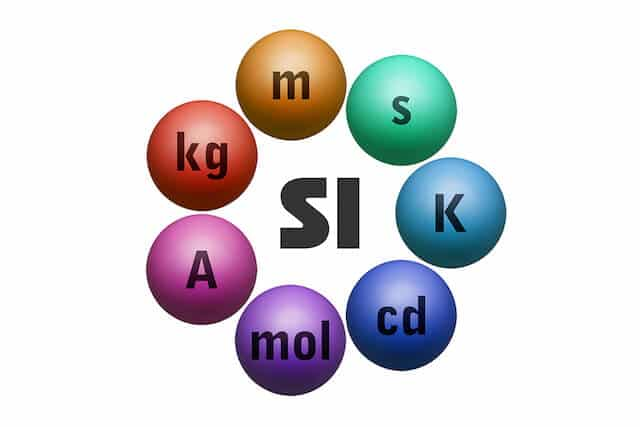SI units, also known as the metric system, are standard definitions of measurements of physical quantities which were defined by the International System of Units (SI, from the French Système international d’unités). Physicists use these units to describe the values of certain variables like time, distance, and mass. Without them, it would be very hard for different scientists to compare and share their experimental results. These units are essential for physics calculations as well because it allows us to define set formulas which relate one variable to others and can be used to derive one variable from others.

A subset of the SI units is the SI base units. These base units constitute the most crucial set of SI units as they can be used to derive every other SI unit. There are 7 of them in total:

 Measurement Unit name SI unit symbol Time second s Mass kilogram kg Length/ Distance metre m Temperature kelvin K Amount of substance mole mol Electric current ampere A Luminous intensity candela cd

Each base unit also has a standard definition which is essential for experimental accuracy in physics. Measurements need to be taken with appropriately calibrated instruments, and the definitions help them to make sure they are standardised across the board. Here are some simplified base unit definitions:

 Unit name Symbol Definition second s 9,192,631,770 periods of the radiation of a Caesium-133 atom. kilogram kg It was previously defined as the mass equal to the mass of 1 dm3 of water at atmospheric pressure and about 4°C. It is now redefined based on the Plank constant, but approximately the same in value. metre m The distance travelled by light in 1/ 299,792,458 of a second. kelvin K A change in thermodynamic temperature that results in a change of thermal energy by 1.380 649×10−23 J. It uses absolute zero as a base (0K), the lowest possible temperature a substance can have. mole mol One mole = 6.02214076×1023 particles (Avogadro’s constant). You can approximate it to 6.02 × 1023 for your exams. ampere A An ampere is the amount of electric current that produces an attractive force of 2.7 × 10–7 Newtons per meter of separation between two wires. candela cd The luminous intensity of a source that emits monochromatic radiation of 540 × 1012 Hz and a radiant intensity of 1/683 watt per steradian in a given direction.

The physical quantities of other units can be determined by combining the base units in specific ways. These units are called derived units. Here are some derived units and how to calculate them from the base units

 Measurement Unit name SI unit calculation Velocity – ms-1 Acceleration – ms-2 Density – kgm-3 Pressure Pascal (Pa) kgm-1s-2 Charge Coulomb (C) As Resistance Ohms (Ω) kgm2s-3A-2 *m-x = 1/mx

As you can see, some variables like density do not have a specific unit name (i.e. density is measured in terms of  kgm-3). However, some derived units do have unique names which are themselves derived from the base units, like pressure (i.e. pressure is measured in terms of Pa, but you calculate Pa from kg, m, and s).

It is also vital for you to know your SI prefixes. These are prefixes and symbols placed in front of other SI units to show that you are referring to that unit, multiplied by a certain number. This is used for convenience when dealing with very small or huge quantities. For example, 0.000001m (metre) would be better represented as 1μm (micrometre). Here are the prefixes that you need to know:

 Prefix name Symbol Value pico p 10-12 nano n 10-9 micro μ 10-6 milli m 10-3 centi c 10-2 deci d 10-1 kilo k 103 mega M 106 giga G 109 tera T 1012

An astute understanding of SI units and prefixes are the hallmarks of a good foundation in physics. It is essential that you know when and how to use each unit, as well as what they mean. If you find yourself in need of help understanding SI units or other physics measurements, you can consider engaging with physics tuition through a private tutor. A dedicated private physics tutor will be able to clearly explain these concepts and more based on your individual learning needs.